# OECD Road accidents data analysis 4 - Regression analysis Death per Habitant on Accident NumberPhoto by Yoksel 🌿 Zok on Unsplash

This post is following of above post.
In the previous post, we see it is better to convert all variables to logarithm to make more normal distribition looking.
So, let's make new variables.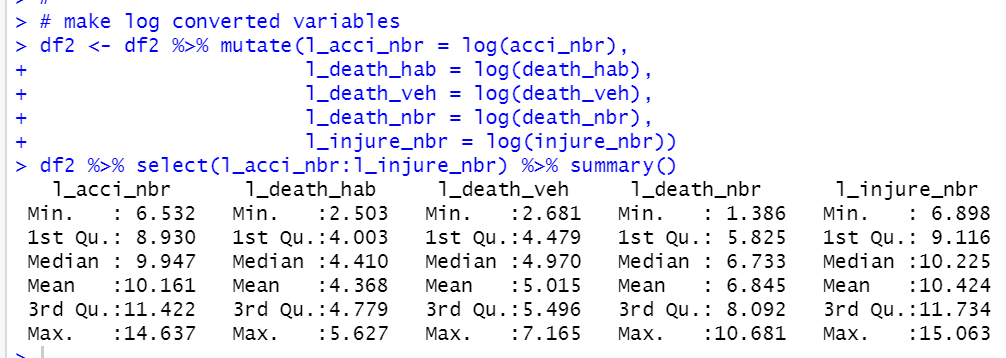Then, let's see scatter plot for l_death_hab and l_acci_nbr.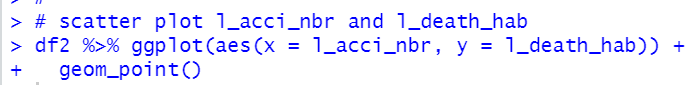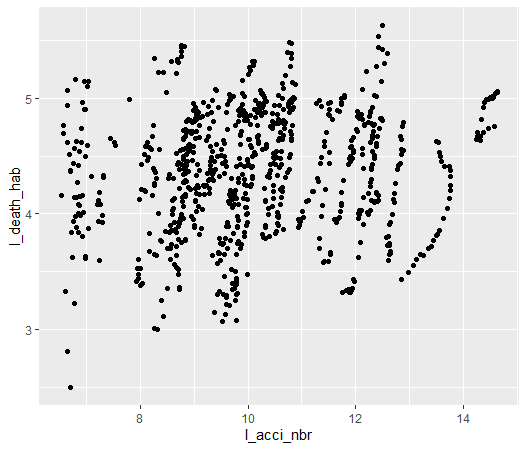Let's do linear regression analysis for l_death_hab on l_acci_nbr.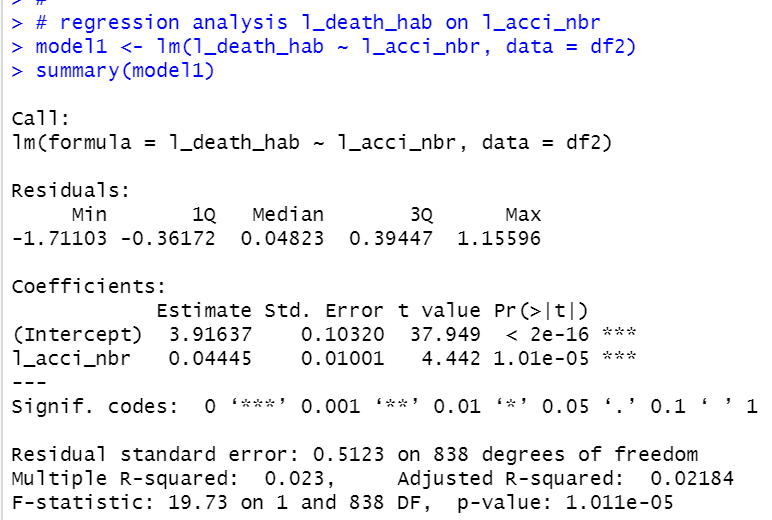We see p-value is 1.011e-05, so this model is statistically significiant.

The coefficient of l_acci_nbr is 0.04445 and it's p-value is 1.01e-05, So, l_acci_mbr is statistically related to l_death_hab.

Multiple R-squared is 0.023, so this model explains l_death_hab value for only 2.3%.

Let's add variable "time" and see how match Multiple R-squared will be inproved.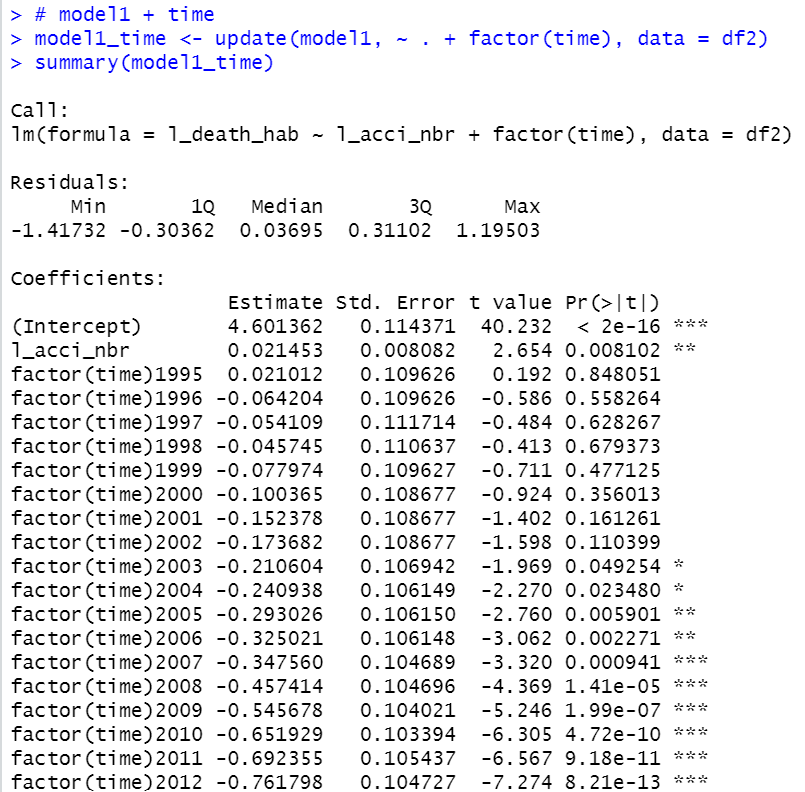Multiple R-squared is 0.3923, so 39% of l_death_hab value is explained by this model.

It is much inproved!

Then, let's add "iso" for explanatory variables.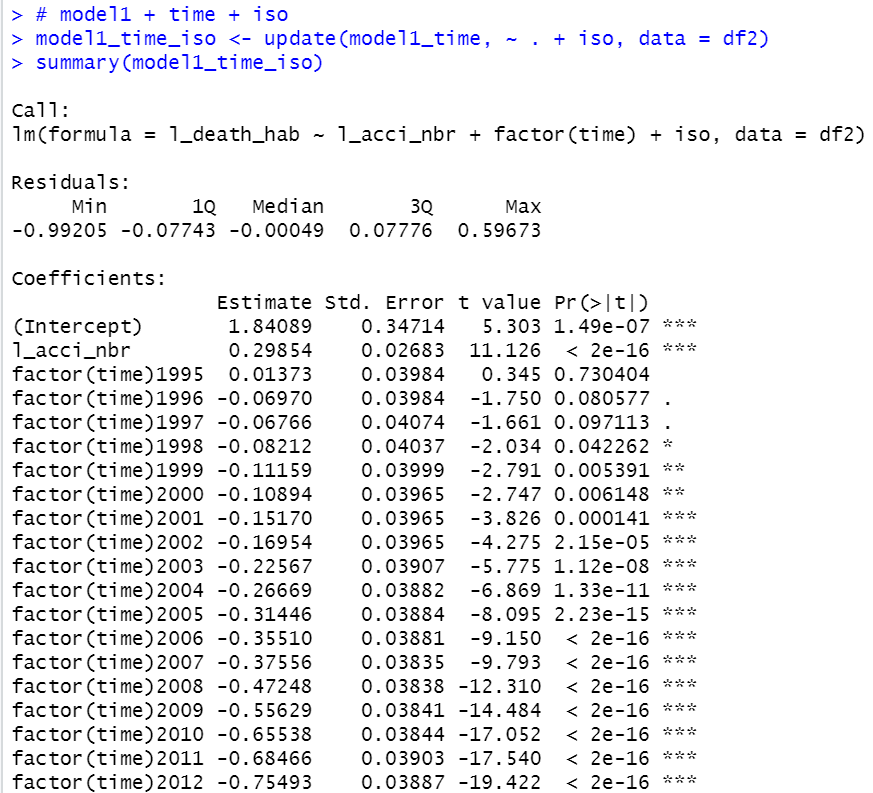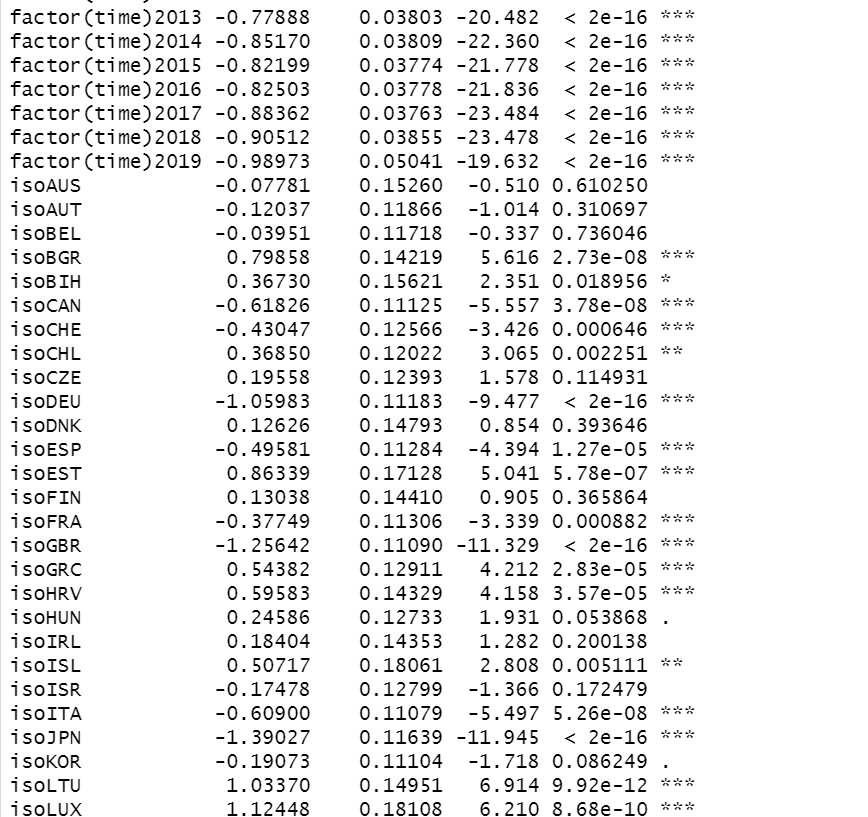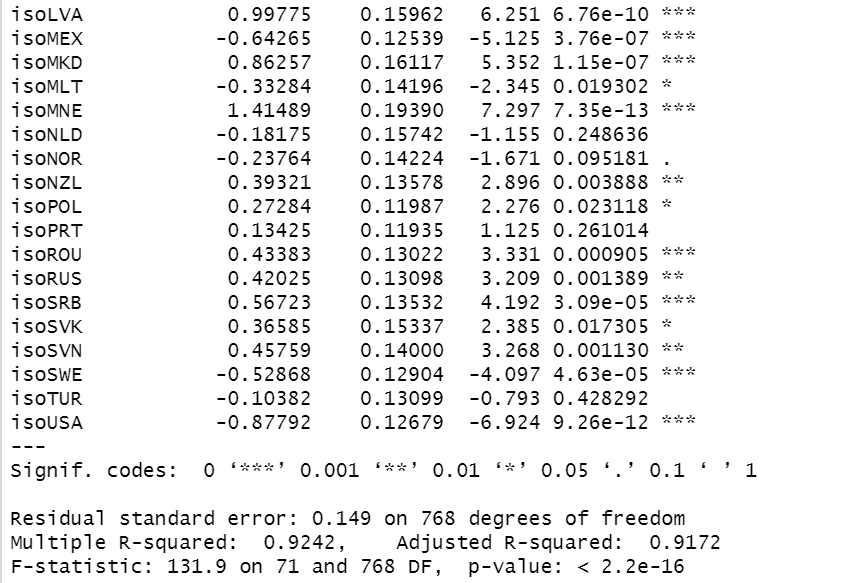Multiple R-squared is 0.9242, so 92% of l_death_hab is explained by this model.
l_acci_nbr coefficient is 0.29854. This means if acci_nbr increased by 1%, death_hab would incread by 0.29854%.

Let's see residual plot.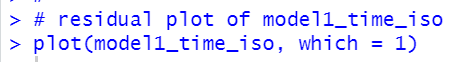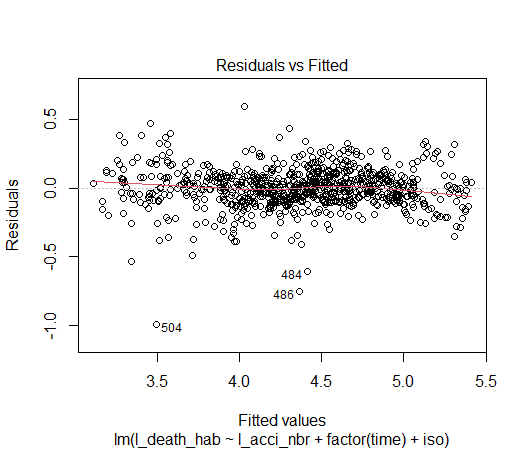It seems there is not heteroskedasticity.

Let's conform it with lmtest library's bptest() function.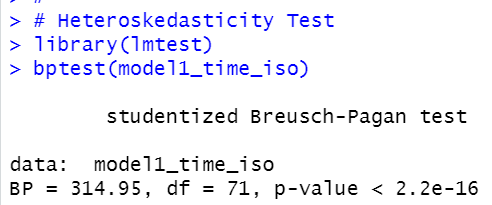Oh, p-value is smaller than 2.2e-16, so this model rejects homoskedacticity.

So, we have to see heteroskedasticity-robust coefficients.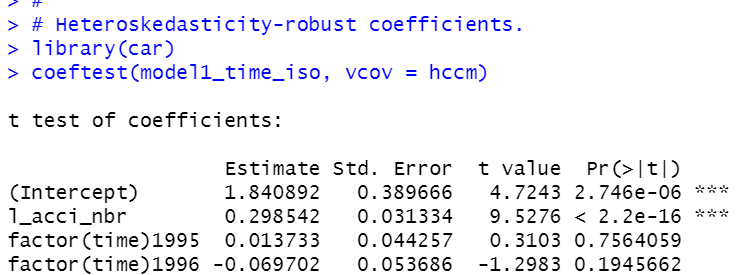We still see l_acci_nbr is statistically significant.

That's it. Thank you!